Next: Born Approximation Up: Scattering Theory Previous: Introduction

# Fundamentals

Consider time-independent, energy conserving scattering in which the Hamiltonian of the system is written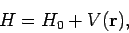(1250)

where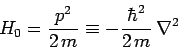(1251)

is the Hamiltonian of a free particle of mass, and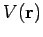the scattering potential. This potential is assumed to only be non-zero in a fairly localized region close to the origin. Let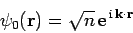(1252)

represent an incident beam of particles, of number density, and velocity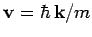. Of course,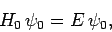(1253)

where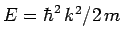is the particle energy. Schrödinger's equation for the scattering problem is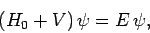(1254)

subject to the boundary condition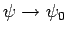as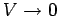.

The above equation can be rearranged to give(1255)

Now,(1256)

is known as the Helmholtz equation. The solution to this equation is well-known: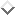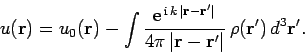(1257)

Here,is any solution of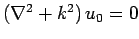. Hence, Eq. (1255) can be inverted, subject to the boundary conditionas, to give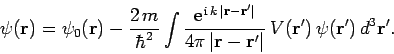(1258)

Let us calculate the value of the wavefunction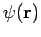well outside the scattering region. Now, ifthen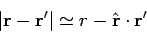(1259)

to first-order in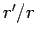, whereis a unit vector which points from the scattering region to the observation point. It is helpful to define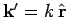. This is the wavevector for particles with the same energy as the incoming particles (i.e.,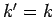) which propagate from the scattering region to the observation point. Equation (1258) reduces to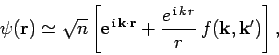(1260)

where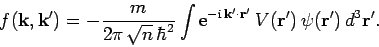(1261)

The first term on the right-hand side of Eq. (1260) represents the incident particle beam, whereas the second term represents an outgoing spherical wave of scattered particles.

The differential scattering cross-section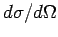is defined as the number of particles per unit time scattered into an element of solid angle, divided by the incident particle flux. From Sect. 7.2, the probability flux (i.e., the particle flux) associated with a wavefunction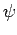is(1262)

Thus, the particle flux associated with the incident wavefunction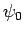is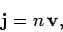(1263)

whereis the velocity of the incident particles. Likewise, the particle flux associated with the scattered wavefunction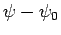is(1264)

where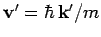is the velocity of the scattered particles. Now,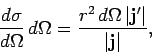(1265)

which yields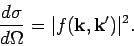(1266)

Thus,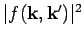gives the differential cross-section for particles with incident velocityto be scattered such that their final velocities are directed into a range of solid anglesabout. Note that the scattering conserves energy, so thatand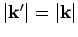.Next: Born Approximation Up: Scattering Theory Previous: Introduction
Richard Fitzpatrick 2010-07-20